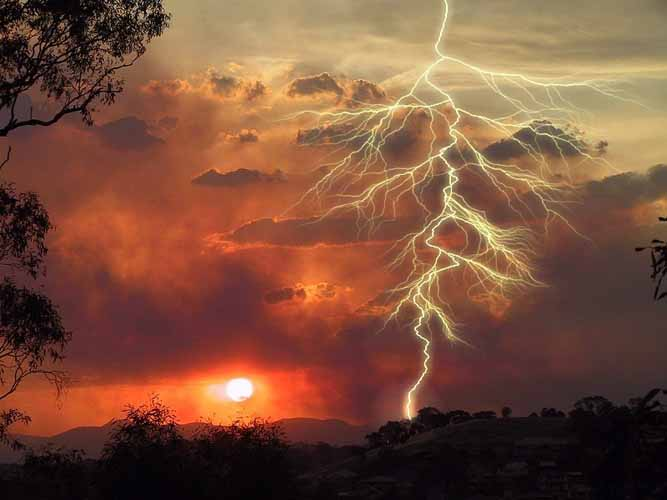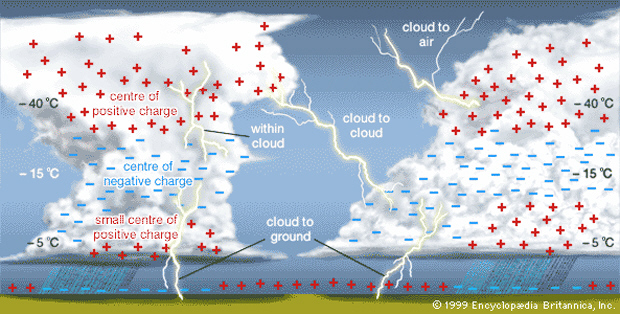#Earth’s Electric Field

The Earth has an electric field.  On average, this field points vertically downwards and it has a magnitude of about 100 N C-1 (Newtons per Coulomb).  It exists because the Earth’s surface carries a negative charge of – 5 x 10C, while the upper atmosphere carries a compensating positive charge.  An average of 400,000 thunderstorms a day sustain a relatively constant electric field.

# The Conductivity of Planet Earth

Electrical conductivity of materials can have values as high as about 108 Ω-1 m-1 for silver (Ag) or copper (Cu), to as low as about 10-18 Ω-1 m-1 for a very efficient insulator.

A knowledge of the conductivity of Earth’s constituent materials is essential to understanding how the Planet interacts with both terrestrial and extra-terrestrial magnetic and electric fields.

Minerals, rocks and soils have conductivities that range over seven orders of magnitude.  Measurements of conductivity and resistance may be used to gather information about subterranean structures of interests to mineral prospectors, archaeologists or forensic scientists.Energy is dissipated in a lightning strike in a way which depends essentially on the conductivity of the ground in the region where it strikes.

#### A current flows from this point up to the thunder clouds above.

The same current must be supplied to the point of the strike by a current flow through the surrounding ground.  Although the current barely lasts a few milliseconds, the situation can be modelled using electrostatics once it is assumed that the ground, at the strike region, has zero conductivity σ and that  the air, away from the path of lightning, is an insulator with zero conductivity.

# Breakdown Field of Humid Air

Any insulator can become a conductor provided that it is placed in a sufficiently high electric field.  That is true for water, air, or the human body…

The minimum field needed to cause this transformation is called the ‘breakdown field’ of the insulator.

### The breakdown field of dry air is about 3 x 106 N C-1.

However, the breakdown field of moist air is only about 1 x 106 N C-1.

Air always contains a few electrons and ionised molecules.  The breakdown of air occurs when the electric field accelerates these charged particles rapidly enough for their collisions with other molecules to produce more electrons and more ionised molecules.

This causes an avalanche of particles that cuts a conducting path through the air and makes sparks fly.

If you have ever been on the receiving end of a static electricity discharge from say… a (presumably “possessed”) car door or a (possibly “haunted”) photocopier, then you know exactly what I mean…  Ouch!!

You have been briefly exposed to an electric field of at least a million newtons per coulomb.

#Lightning Strikes

Since the Earth’s atmosphere is not a perfect insulator, the Earth’s electric field drives a small current downwards.  This would be enough to neutralise the Earth’s negative charge and remove its electric field if it were not for the effects of lightning.

Below a thundercloud, the electric field points vertically upwards and is 50 times stronger than normal.  Enough to make your hair stand on end!

Perhaps even more striking… (Ahem!)

Lightning does not fall to the ground.

### Lightning conducts currents upwards, from the ground to the cloud.

Over the entire surface, about 400,000 thunderstorms a day keep the Earth negatively charged, thus maintaining a relatively constant electric field.

## Equations for the Potential V of a Thunder Strike

Now, let’s try and figure out the potential V on the Earth’s surface at a distance r from the bolt.  With spherical coordinates having their origin at the strike point, the symmetry of the problem indicates that the current density and electric field within the ground are radial, depending on r only.

Within the ground, the electric field is$\boldsymbol {E(r)} = E_{r(r)} \boldsymbol {e_r} = \frac {1}{\sigma} J_r(r) \boldsymbol{e_r}$

The current density can be described in terms of$J(r) = \sigma {E_r(r)} = - \frac {I} {2 \pi {r^2}}$

At large distances, the potential is zero.

At a radius r, the potential is$V(r) = - \int_\infty ^r {E_r(r') dr'} = + \frac {I} {2 \pi \sigma} \int_\infty ^r \frac {dr'}{dr'^2} = - \frac {I} {2 \pi \sigma} \left [ \frac {1}{r'} \right ] _\infty ^r = - \frac {I} {2 \pi \sigma r}$

For example, a stroke of lightning carrying a charge of 20 Coulombs, lasting about 2 milliseconds (2.0 x 10-3 s), occurs at a point on the ground where the conductivity is 0.010 Ω-1 m-1.

Using the conductivity equation, we obtain$\sigma E = \frac{I}{2 \pi r^2}$

And so we see that the radius is$r = \sqrt {\frac{I}{2 \pi \sigma E}} = \sqrt {\frac{\Delta Q}{2 \pi \sigma E \Delta t}}$

The distance at which the field acts 100 V m-1 is equal to$r \sqrt {\frac{20 C}{2 \pi \times 10^{-2} \Omega^{-1} m^{-1} \times 100 V m^{-1} \times 2.0 \times 10^{-3} s}} = 40 metres$

At this distance, the potential V is$V = - \frac{I}{2 \pi \sigma r} = - \frac{20 C}{2 \pi \times (2.0 \times 10^{-3} s \times 10^{-2} \Omega^{-1} m^{-1}) \times 40 m} = - 4.0 \times 10^3 V$

Even though the magnitude of the electric field is only 100 V m-1, 40 metres away from a lightning strike, the potential is – 4 kV (kiloVolts).

# How Can Lightning Hurt You?

Here’s how it works: A lightning strikes hits a primary object, then the current disperses through the ground until it dissipates.

Research shows that it is hazardous out to a radius of 10 metres.  However, people have sustained injuries up to a radius of 15 to 30 metres away.We often think of a direct strike that can injure or even kill someone.  Mostly at risk are field workers, hill-walkers, golfers… but a direct strike only accounts for 3-5% of casualties.

According to Environment Canada, the dangers of lightning can also arise from a number of secondary effects:

• Ground Current (40-50%) – Lightning enters the earth, travels through it and voltages are set up in the ground. One part of your body contacts one voltage, and another part of your body contacts a different voltage. The difference in voltage is what drives the current through your body.  High current surface arcs are associated with ground currents.  Typically, they appear as bright arcs of light radiating from a strike point like spokes of a wheel, in the air above the ground’s surface.
• Side Flash (20-30%) – When lightning that has hit an object travels partly down the object before jumping to a nearby victim.  Never seek shelter near a lone tree or other tall object, as a side flash can splash lightning from these tall objects onto a nearby object to reach the ground.
• Contact (15-25%) – The person is touching or holding onto an object which lightning attaches itself to, such as wire fencing, telephones poles, etc. When inside a house, either a corded telephone or water pipe such as a faucet can also cause this.
• Upward Leaders (10-15%) – Or streamers, are currents of positive charges that start growing upward from the ground from elevated objects. They are produced in response to the step leaders coming down from the cloud base.  If a downward step leader and a streamer meet, a conductive path forms and a lightning strike occurs.  Other streamers in the area of the main stroke still carry a charge.  This charge is much smaller than the main stroke but still large enough to cause injury or death to humans.

So… Always Beware Out There!  😮

Still want to know more about lightning?

Even more on this spectacular phenomenon of Nature when we discover all about sprites, elves and gnomes.  That’s right!  Soon to follow…

## One thought on “Lightning and the Earth’s Electric Field”

1.M> Rfart says:

Dear Sirs

In regard to earth magnetic field(s) , As I understand there is magnetic flux flowing from north pole to south pole in circle 3D lines surrounding the earth’s crust , however, there is also magnetic field raised from earth’s crust materials & there is sunlight’s magnetic field too , therefore the magnetic flux flowing from north pole to south pole should be in wave formats – modulated by the sunlight & earth crust’s field(s) ,.
I am looking for to find out how these wave forms are looks like ?, what is their formats ? , what are the frequencies of each type ?,.

appreciate your kindness assistance in this regard, it’ll be great help for my project indeed ,

Thank you so much

Kind Regards
Meir Efrat